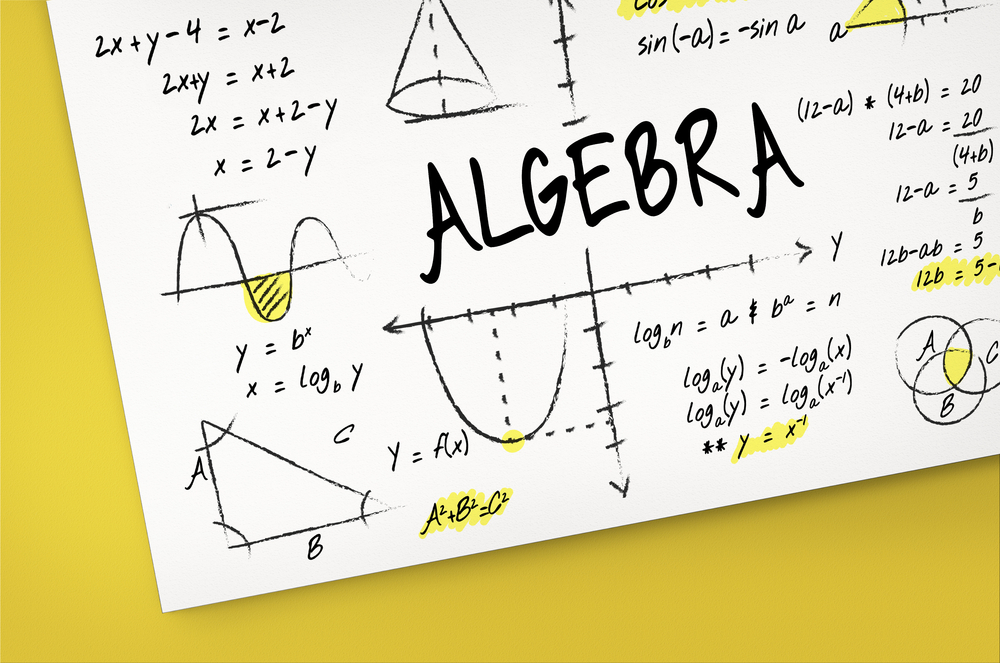# Learning Algebraic Formula | The Formula of (a-b)²The algebra formula, (a-b)^2, is used to calculate the square of a binomial. It is also called the formula used to calculate the square of the difference of 2 identities or terms. We have covered the formula and a solved example for your better understanding of the formula of (a-b)². So, let’s dive in:

## Algebra Formula: (a-b)^2 Proof

Now, the formula is:

(a－b)²＝a²－2ab＋b²

But if you are not sure about it, here is how you can prove it:

(a－b)²＝(a－b)(a－b)

⇒ a(a－b)－b(a－b)

⇒ a²－ab－ab＋b²

⇒ a²－2ab＋b²

𝑎+ 2𝑎𝑏 + 𝑏2

P=2(a+b)

## A Solved Example

Find out the value of  (2a – 3b)2

#### Solution

(2a – 3b)2 = (2a)2 + (3b)– 2(2a)(3b)

= 4a2 + 9b2 – 2(2a)(3b)

= 4a2 + 9b2 – 12ab

Therefore,

(3a – 2b)2 = 4a2 + 9b2 – 12ab

And that’s the formula of (a-b)², explained and solved, for you.

### Find Top Tutors in Your AreaWith over 3 years of experience in teaching, Chloe is very deeply connected with the topics that talk about the educational and general aspects of a student's life. Her writing has been very helpful for students to gain a better understanding of their academics and personal well-being. I’m also open to any suggestions that you might have! Please reach out to me at chloedaniel402 [at] gmail.com